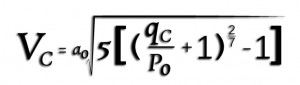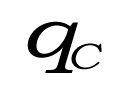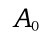# Pitot Static System…Airspeed Calculation

Pitot Static System…Airspeed Calculation

A.  Airspeed Calculation:

Airspeed is calculated as a function of the difference between Pitot Pressure and Static Pressure as follows:

Calculated or Indicated airspeed is indicated airspeed corrected for instrument errors, position error (due to incorrect pressure at the static port) and installation errors.

Calibrated airspeed values less than the speed of sound at standard sea level (661.4788 knots) are calculated as follows:minus position and installation error correction.

Whereis the impact pressure (inches Hg) sensed by the pitot tube,is 29.92126 inches Hg; static air pressure at standard sea level,is 661.4788 knots:, speed of sound at standard sea level

Units other than knots and inches of mercury can be used, if used consistently.

This expression is based on the form of Bernoulli’s equation applicable to a perfect, incompressible gas. The values forandare consistent with the ISA i.e. the conditions under which airspeed indicators are calibrated.

Keep in mind that this is for your basic vanilla airspeed indicator and does not include calculations for TRUE Airspeed for which you must include the variables of True Temperature and True Altitude.

Stay tuned for upcoming Blogs